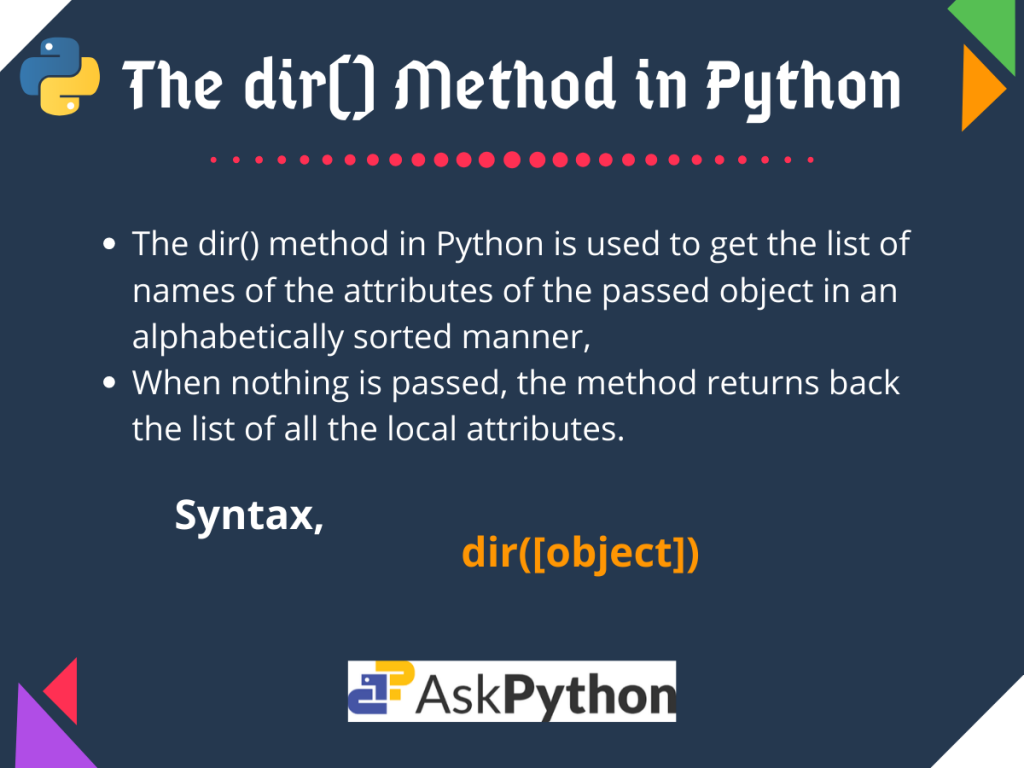# Quick Overview of the Python dir() MethodToday we are going to discuss the Python dir() method.

So let’s get started.

## The Python dir() Method Basics

The `dir()` method in Python is widely used to get the list of names of the attributes of the passed `object` in an alphabetically sorted manner.

```dir([object])
```

Here, `object` is an optional argument. When any Python object is passed to the `dir()` method, it returns a list containing all the attributes of that object. And when nothing is passed, the method returns back the list of all the local attributes.

For objects with defined `__dir__()` method, the `dict()` leads to the call for it and hence should return a list of attributes related to the object.

### Python dir() Example

Now that we have a basic idea of the `dir()` method, let us take a look at an example to have a better understanding.

```#empty dir()
print("dir() :", dir())

#list initialisation
list1 = ['name', 'class', 'roll']

#dictionary initialisation
dict1 = {0: 'bad', 5: 'fair', 10: 'good'}

print("\ndir(list1) :", dir(list1))
print("\ndir(dict1) :", dir(dict1))
```

Output:

```dir() : ['__annotations__', '__builtins__', '__cached__', '__doc__', '__file__', '__loader__', '__name__', '__package__', '__spec__']

dir(list1) : ['__add__', '__class__', '__contains__', '__delattr__', '__delitem__', '__dir__', '__doc__', '__eq__', '__format__', '__ge__', '__getattribute__', '__getitem__', '__gt__', '__hash__', '__iadd__', '__imul__', '__init__', '__init_subclass__', '__iter__', '__le__', '__len__', '__lt__', '__mul__', '__ne__', '__new__', '__reduce__', '__reduce_ex__', '__repr__', '__reversed__', '__rmul__', '__setattr__', '__setitem__', '__sizeof__', '__str__', '__subclasshook__', 'append', 'clear', 'copy', 'count', 'extend', 'index', 'insert', 'pop', 'remove', 'reverse', 'sort']

dir(dict1) : ['__class__', '__contains__', '__delattr__', '__delitem__', '__dir__', '__doc__', '__eq__', '__format__', '__ge__', '__getattribute__', '__getitem__', '__gt__', '__hash__', '__init__', '__init_subclass__', '__iter__', '__le__', '__len__', '__lt__', '__ne__', '__new__', '__reduce__', '__reduce_ex__', '__repr__', '__setattr__', '__setitem__', '__sizeof__', '__str__', '__subclasshook__', 'clear', 'copy', 'fromkeys', 'get', 'items', 'keys', 'pop', 'popitem', 'setdefault', 'update', 'values']
```

As you can see, here we have at first passed nothing, then a list object, and finally a dictionary object to the `dir()` method and have printed out the returned list.

From the above output, we can clearly see the different attributes available for the list and dictionary objects. For the case where nothing is passed to the function, we get all the names of the methods or attributes in the local scope.

## Working with the dir() Method in Python

So now let us try out some more examples where we try to use the `dir()` function on objects of user-defined classes as well as ones with defined `__dir__()` method.

### 1. With Custom Objects

Now that we have applied the `dir()` method for built-in classes like lists and dictionaries. Let us try finding out the results for custom objects of a user-defined class with undefined `__dir__()`.

```#class
class shape:
name = "rectangle"
sides = 4

obj = shape()
print(dir(obj)) #dir for our custom object
```

Output:

```['__class__', '__delattr__', '__dict__', '__dir__', '__doc__', '__eq__', '__format__', '__ge__', '__getattribute__', '__gt__', '__hash__', '__init__', '__init_subclass__', '__le__', '__lt__', '__module__', '__ne__', '__new__', '__reduce__', '__reduce_ex__', '__repr__', '__setattr__', '__sizeof__', '__str__', '__subclasshook__', '__weakref__', 'name', 'sides']
```

Here, `obj` is an object of the `shape` class with name rectangle and sides = 4. Passing this obj object to the `dir()` method, we get the above set of attributes.

Note, this list includes the `name` as well as the `sides` variable too.

### 2. With defined __dir__()

As mentioned earlier in this article, for objects with defined `__dir__()` method, the `dir()` method calls the corresponding `__dir__()` method which must again return a list of attributes.

Let us try to understand that with an example.

```#class
class shape:
name = "rectangle"
sides = 4
def __dir__(self):
return ['Square','Circle']

obj = shape()
print(dir(obj)) #dir for our custom object
```

Output:

As you can see, for the object `obj` of the shape class, the `__dir__()` method is called and the above list of attributes is returned at the site of `dir(obj)` call.